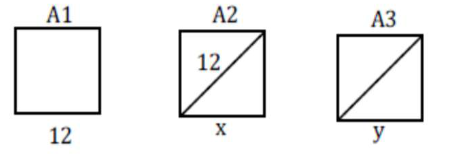# Prove the followingQuestion:

Let $A_{1}, A_{2}, A_{3}, \ldots \ldots$ be squares such that for each $n \geq 1$, the length of the side of $A_{n}$ equals the length of diagonal of $\mathrm{A}_{n+1}$. If the length of $\mathrm{A}_{1}$ is $12 \mathrm{~cm}$, then the smallest value of $\mathrm{n}$ for which area of $\mathrm{A}_{n}$ is less than one, is

Solution:$x=\frac{12}{\sqrt{2}} \quad y=\frac{12}{(\sqrt{2})^{2}}$

$\therefore$ Side lengths are in G.P.

$\mathrm{T}_{\mathrm{n}}=\frac{12}{(\sqrt{2})^{\mathrm{n}-1}}$'

$\therefore \quad$ Area $=\frac{144}{2^{n-1}}<1 \quad \Rightarrow 2^{n-1}>144$

Smallest $\mathrm{n}=9$